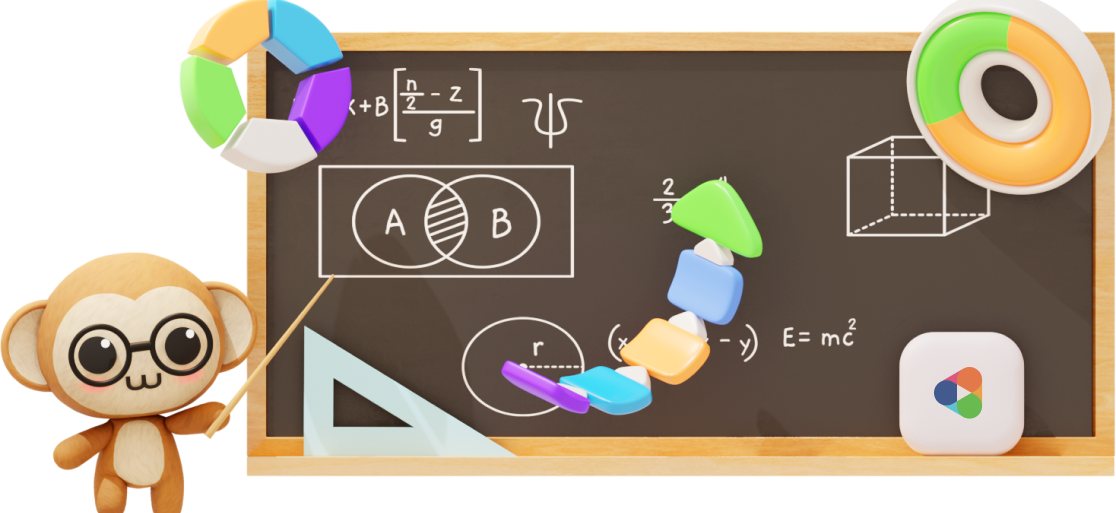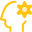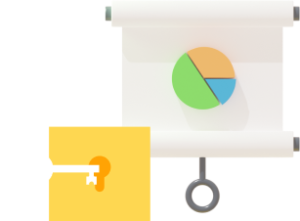# Primary 4 Syllabus

Aligned with the latest 2021 Singapore MOE Math syllabus and endorsed by Education Alliance of Finland.
Hundreds of hours of learning content for all topics covered in P4 to get your child build a strong Math foundation!## Overview

In P4, your child will expand their problem-solving toolbox by learning heuristics, such as drawing diagrams and looking for patterns.

If your child’s foundation is weak, they will struggle. Soon, they dread doing their homework or going to class.

They are afraid that they can’t keep up.

With Practicle, they can!

First, our platform will use AI to personalise our questions to match your child’s current ability.

Then, over time, as your child improves, we’ll give them tougher questions.

Even if the class has moved on, they can continue to build their skills.

That’s the beauty of self-paced learning.

Our syllabus is 100% aligned with MOE and is certified by the Education Alliance of Finland (EAF) for educational quality and effectiveness.

### Features##### Gamification to make learning engaging and rewarding##### A.I. + Practicle system to personalise questions to your child’s ability## Overview of Primary 4 Math Syllabus

Here are the P4 topics and skills that are covered our system. The 2020 Primary 4 Math Syllabus can be divided into 3 main branches – Numbers, Measurement and Geometry and finally, Statistics.

#### P4 Math: Numbers

The Primary 4 (P4) topics that are covered under Numbers are Whole Numbers, Multiplication, Division, Factors and Multiples, Fractions and Decimals. The following shows the breakdown of the skills in topic:#### Whole Numbers

• Compare and order numbers
• Understand place values up to 10 000
• Round numbers to the nearest 10, 100 and 1 000
• Estimate the value of an equation#### Multiplication

• Multiply a 4-digit number by 1-digit
• Multiply up to 3-digits by 2 digits
• Multiply up to 3-digits by 10#### Division

• Divide a 4-digit number by 1-digit#### Factors & Multiples

• Check if a 1-digit number is a factor of a given number within 100
• Find common factors between 2 numbers
• Check if a number is a multiple of a given 1-digit number
• Find common multiples of 2 1-digit numbers#### Fractions

• Compare and order whole numbers and fractions
• Compare fractions with a common denominator
• Add fractions with different denominators
• Subtract fractions with different denominators
• Find a part of a set of objects
• Convert mixed numbers to improper fractions and vice versa#### Decimals

• Understand place values of tenths
• Understand place values of hundredths
• Understand place values of thousandths
• Convert a decimal to a fraction and vice versa
• Compare and order decimals
• Rounding decimals to the nearest whole number, 1 decimal place and 2 decimal places#### Operation of Decimals

• Add decimals up to 2 decimal places
• Subtract decimals up to 2 decimal places
• Multiply decimals up to 2 decimal places by 1-digit
• Divide decimals up to 2 decimal places by 1-digit
• Divide a whole number by a whole number

### P4 Math: Measurement and Geometry

The Primary 4 (P4) topics that are covered under Measurement and Geometry are Time, Angles, Line symmetry, 2D Shapes, Area and Perimeter. See the following for the breakdown of skills in each topic:#### Time

• Find the duration of an event
• Measure time in seconds
• Find starting time / ending time#### Lines

• Identify symmetric figures
• Check if a straight line is a line of symmetry
• Complete a symmetric figure with respect to a given line of symmetry#### Angles

• Name an angle
• Measure angles in degrees
• Relate quarter, half 3/4 and complete turns to angles in degrees
• Find angles between 2 directions on a 8-point compass#### Area & Perimeter

• Find the length of a side of a square
• Find the dimension of a rectangle
• Find the area of rectilinear figures#### 2D Shapes

• Know the properties of a square
• Know the properties of a rectangle

### P4 Math: Graphs

The Primary 4 (P4) topics that are covered under Statistics include Data Analysis. Refer below for the breakdown of the skills in this topic.#### Data Analysis

• Complete a table with given data
• Read and interpret line graphs

### Sample P4 questions and video explanations### Experience Practicle free, no commitment no hidden cost no lock-in

Try our learning platform free with a 7 day trial
and see if your child likes it.